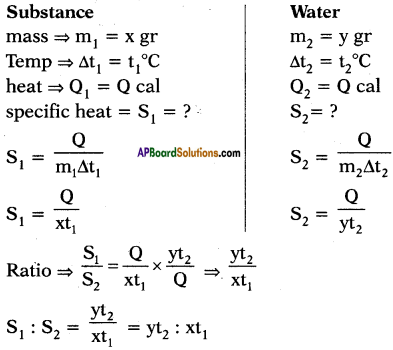AP State Board Syllabus AP SSC 10th Class Physical Science Important Questions Chapter 1 Heat.

## AP State Syllabus SSC 10th Class Physics Important Questions 1st Lesson Heat

### 10th Class Physics 1st Lesson Heat 1 Mark Important Questions and Answers

Question 1.
What is humidity? (AP March 2015)
Humidity :
The amount of water vapour present in air is called humidity.

Question 2.
Define latent heat of Fusion. (AP Morch 2016)
Latent heat of Fusion :
At constant temperature, the heat energy required to convert one gram of solid completely into liquid is called latent heat of Fusion.Question 3.
While drinking water, Rama spilled some water on the floor. After some time, the water disappeared from the floor. What happened to the water? (TS June 2015)

• The water disappeared due to evaporation because we know that as the surface area increases rate of evaporation also increases.
• So water molecules escape from the floor to air.

Question 4.
Give an example to explain that evaporation is a cooling process. (TS March 2016)
The examples to explain that evaporation is a cooling process are

1. Drying of wet clothes
2. When the floor is washed with water, the water on the floor disappears.
3. Sweating, etc.

Question 5.
Let heat is not lost by any other process between two objects in thermal contact, “Net heat lost (by hot body) = Net heat gain (by cold body).” above statement indicates a principle. Write the name of that principle. (AP March 2019)
Principle of method of mixtures.

Question 6.
Convert 25°C into Kelvin scale. (AP SCERT: 2019-20)
25°C = (273 + 25) K = 298 K

Question 7.
Given a beaker with water, a thermometer and a stand, draw the arrangement of an experiment to measure boiling point of water. (AP SA-1:2019-20)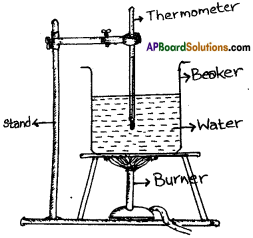Question 8.
Define heat.
Heat: Heat is a form of energy which is transferred from one body to the other body due to the difference in their temperature.

Question 9.
What is meant by thermal equilibrium?
Thermal Equilibrium :
Two bodies are at the same temperature then they are said to be in thermal equilibrium.Question 10.
Define dew.
Dew :
The water droplets condensed on surface are known as dew.

Question 11.
What is boiling?
Boiling is a process in which the substance changes from liquid to gas.

Question 12.
What is melting?
Melting :
The process in which the substance changes from solid to liquid state is called melting.

Question 13.
What are the different energies possessed by system (body or material)?

1. Linear kinetic energy
2. Rotational kinetic energy
3. Vibrational energy
4. Potential energy and Internal energy (I.E).

Question 14.
Why does samosa seem to be cool but hot when we eat?
Because the curry inside samosa contains ingredients with higher specific heats.

Question 15.
On which factors does rate of evaporation depend?

1. Surface area
2. temperature
3. the amount of vapour already present in the surrounding air.

Question 16.
What is the value of latent heat of vapourization of water?
Latent heat of vapourization of water = 540 cal/gm. (or) 2.26 × 106 J/kg

Question 17.
What is the value of latent heat of fusion of ice?
Latent heat of fusion of ice is 80 cal/gm. (or) 3.26 × 105 J/kg

Question 18.
Give some liquids which solidify (convert into solid) in winter season.
Coconut oil, ghee are some liquids which solidify in winter season.

Question 19.
What is freezing?
Freezing :
The process in which the substance changes from liquid to solid state by losing some energy from it is called freezing.Question 20.
Which will have lower temperature when we take out a wooden piece and a metal piece from a fridge?
The metal piece will have lower temperature as compared to the wooden piece when they are taken out of the fridge.

Question 21.
When do you say there is thermal equilibrium between two bodies?
It is said that there is thermal equilibrium between two bodies when there is no transfer of heat energy between them.
(OR)
When temperature between two bodies is same it is said that there is thermal. equilibrium between them.

Question 22.
What is absolute temperature?
Temperature measured in Kelvin scale is called absolute temperature.

Question 23.
What is latent heat of vapourisation?
At constant temperature the heat energy required to change one gram of liquid into gaseous state.

Question 24.
What is boiling point?
The temperature at which the substance changes from liquid to gaseous state at the fixed temperature is called boiling point.

Question 25.
What is melting point?
Melting point :
The temperature at which the substance changes from solid to liquid state at constant temperature is called melting point.

Question 26.
How is aquatic animal able to live at poles?
The ice has less density compared to water. So it forms a layer on the top of water which prevents the solidification of water.

Question 27.
What are the phases of water present at 0° C?
Two phases namely, ice and water.

Question 28.
What happens if external pressure of liquid increases?
The boiling point of the liquid will increase.

Question 29.
Does ice melt below 0° C?
Yes, if the external pressure increases it melts at low temperature.Question 30.
What happens when two objects of same temperature are in contact with each other?
Heat does not flow between two objectives.

Question 31.
What is the principle involved in pressure cooker?
Boiling point of liquid increases with external pressure.

Question 32.
What happens to kinetic energy of particles if we increase the temperature?
Kinetic energy of particles increases with increase of temperature.

Question 33.
Why does transfer of heat energy take place between systems?
When heat energy gives to the system, internal energy increases. Similarly, internal energy decreases when heat energy flows out of the system.

Question 34.
What is internal energy?
Internal energy :
It is the energy possessed by the system by virtue of its molecular motion and molecular configuration. It is a stored energy. It depends on the temperature of the system.

Question 35.
What is transit energy?
Transit energy:
Energy possessed by a system which can cross its boundary is called transit energy. Heat and work are transit energies.

Question 36.
Where does air get? vapour from?
The vapour may come from evaporation of water from the surfaces of rivers, lakes, ponds, and from the drying of wet clothes, sweat, and so on.

Question 37.
Why do pigs toil in the mud during hot summer days?
They do not have sweat glands for evaporation process. So pigs toil in the mud.

Question 38.
Why is it easy to cook food in a pressure cooker?
We know as the atmospheric pressure increases the boiling point of water increases. So we can increase the boiling point of water to 120°C in a pressure cooker. So it is \ easy to cook in a pressure cooker.Question 39.
Why is water used as coolant?
Water has the highest specific heat. So it takes lot of time to become hot. So it is used as coolant.

Question 40.
How is fog formed?
The water molecules present in vapour condense on the dust particles in air and form small droplets of water which form a thick mist called fog.

Question 41.
Equal amounts of water is kept in a cup and in a disc. Which will evaporate faster? Why?
The water present disc evaporates faster because of greater surface area.

Question 42.
Explain why dogs pant during hot summer days using the concept of evaporation.
Dog does not have pores on its body. The only place where a dog can sweat is on its foot pads and the rest of the body is covered in a fur coat. So it cannot sweat; that’s why dogs pant to keep cool themselves.

Question 43.
Same amount of heat is supplied to two liquids A and B. The liquid A shows a greater rise in temperature. What can you say about the specific heat of A?
The specific heat of A is less than that of B because rise in temperature is inversely proportional to temperature.

Question 44.
What is the specific heat of-water at boiling point?

1. Generally, the specific heat of water is 1. (or) 4.187 KJ / Kg K
2. Specific heat of water at 100° C = 4.219 KJ / KgK

Question 45.
What is the equation of heat energy when change the state?
Q = mL
Where m = mass of body, L = latent heat.

Question 46.
Convert 212°F into Kelvin scale.
212°F= 100°C. So 100 + 273 = 373 K.

Question 47.
Convert 310 K into centigrade system.
310-273 = 37°C.Question 48.
Are the processes of evaporation and boiling the same?
No. Evaporation takes place at any temperature, while boiling occurs at a definite temperature called the boiling point.

Question 49.
Define latent heat of vaporization?
The heat energy required to change one unit mass of liquid to gas at constant temperature is called latent heat of vaporization.
$$L=\frac{Q}{m}$$
The value of latent heat of vapourization of water is 540 cal/gm.

Question 50.
What is meant by internal energy?
Combination of linear kinetic energy, rotational kinetic energy, vibrational energy, and potential energy of molecules is known as internal energy of the system.

Question 51.
Write the formula for resultant temperatures of a mixture, when V1 ml of water at T1°C is mixed with V2 ml of water at T2° C.
Resultant temperature $$T=\frac{V_{1} T_{1}+V_{2} T_{2}}{V_{1}+V_{2}}$$

Question 52.
Write the equation of heat energy when change the temperature.
Q = mS∆T
m == mass, S = specific heat, AT = change in temperature

Question 53.
The figure shows change in state of ice from – 5°C to 110°C with temperature. What are the melting and vaporization curves?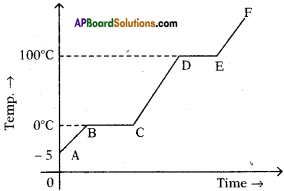BC = melting
DE = vaporization curve.

Question 54.
Write principle of method of mixtures.
When two or more bodies are brought into thermal contact, then heat lost by hot body is equal to heat gain by cold body. Until they attain thermal equilibrium.

Question 55.
Evaporation is a cooling process. Why?
During evaporation process, the energy of the molecules inside the liquid decreases and they slow down.Question 56.
Which factors are influence the rate of evaporation of a liquid?
Rate of evaporation of a liquid depends on surface area, temperature, pressure, and amount of vapour present in surrounding air.

Question 57.
What is meant by fog?
The droplets keep floating in the air and form a thick mist which restricts visibility. This thick mist is called fog.

Question 58.
Why do we sweat while doing a work?
When we work our body produces heat. As a result, the temperature of the skin becomes higher and the water in the sweat glands starts evaporating. This evaporation cools the body.

Question 59.
A samosa appears to be cool when touched outside but it is hot when we eat it. Why?
A samosa appears to be cool outside but it is hot when we eat it because the curry inside the samosa contain ingredients with higher specific heats. Hence they remain hot for a long time.

Question 60.
Equal amounts of water are kept in a cup and in a dish. Which will evaporates faster? Why?
Dish evaporates faster, because dish has large surface area. Evaporation of liquids depends on surface area.

Question 61.
Why water is used as coolant in the cooling system of automobile engines?
Due to high specific heat, water absorbs large amount of heat and temperature does not rise quickly. So water used as coolant in cooling system of automobile engines.

Question 62.
Why do pigs toil around in the mud?
Pigs do not have sweat glands. Water in the mud evaporates and helps the pig to be cool from heat. So pigs toil in the mud during summer.Question 63.
Take small glass bottle with a tight lid. Fill it with water completely without any gaps and fix the lid tightly in such a way that water should not come out of it. Put the bottle into the deep freezer for a few hours. Take it out from the fridge. You observe the glass bottle is broken. Why?
We know, the volume of the water poured into the glass bottle is equal to the volume of the bottle. When the water freezes to ice, the bottle is broken. Because the volume of the ice is greater than the volume of the water filled in bottle.

Question 64.
From the given figure, in which the thermometer mercury level is increases and decreases?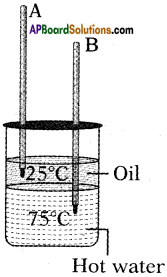Thermometer A(in oil) is increases.
Thermometer B(water) is decreases.

Question 65.
What are the materials are used in to find the specific heat of solid?
Calorimeter, thermometer, stirrer, water steam heater, wooden box, and lead shots.

Question 66.
What is the value of following temperatures in Kelvin scale?
(a) 30° C b) 70° C
a) 30° C = 30 + 273 = 303 K
b) 70° C = 70 + 273 = 343 K

Question 67.
How much heat energy is required to raise the temperature of unit mass of material by 1° C?
1 cal/g – °C = lk cal / kg – K = 4.2 x J/kg – K = 4.2 kJ/kg – K.

Question 68.
How much energy is required to turn 1 g of ice of 0°C into 1 gm of water at 0°C?
The energy required to convert 1 g of ice at 0°C into lg of water at 0°C is latent heat of fusion that is 80 cal/g.

Question 69.
What is the temperature of mixture if 10 g of steam at 100°C is mixed with 50 g of ice at 0°C?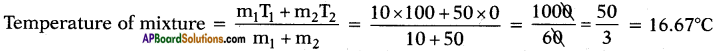Question 70.
Boiling water at 100°C and cold water at t°C are mixed in the ratio of 3 : 5 and the resultant temperature is 40°C. Find the value of t.
Suppose the quantities of water is 3x and 5x.
Given that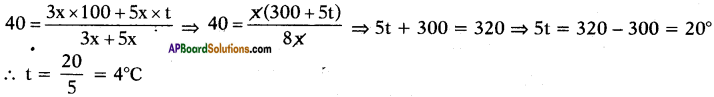Question 71.
What amount of ice can be melted by 4000 cal of heat?
Latent heat of fusion of ice Lf = 80 cal/g
Given that Q = 4000 cal
Q = mLf ⇒ 4000 = m × 80
∴ m = $$\frac{4000}{80}$$ = 50 g

Question 72.
5 gm of ice is at (J°C. It is converted into water at same temperature. How much heat energy is required?
In change the state Q = mL
m = 5 gm, L = Latent heat of fusion = 80 Cal/gm
Q = mL = 5 × 80 = 400 cal.Question 73.
What would be the final temperature of mixture 50 g. of water at 20°C and 50 gm of water at 40°C?
If masses are equal, then resultant temperature of mixture = $$\frac{\mathrm{T}_{1}+\mathrm{T}_{2}}{2}$$
∴ T = $$\frac{20+40}{2}$$ ⇒ T =30

### 10th Class Physics 1st Lesson Heat 2 Marks Important Questions and Answers

Question 1.
Observe the following table regarding the values of specific heat of substances and answer the following questions : (AP SA-I: 2018-19)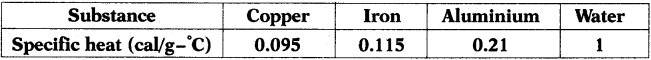i) Which material is suitable as the base of the cooking vessel?
Copper. Because, it has low specific heat value.

ii) Why do we prefer water as a coolant?
Due to high specific heat value of water, it can take more time to heat itself and acts as a coolent.

Question 2.
Why do water drops (dew) form on flowers and grass during morning hours of winter season? (AP March 2016)
During winter nights, the atmospheric temperature goes down. The flowers, grass, etc. become still colder. The air near them becomes saturated with vapour and condensation begins. The water droplets condensed on such surfaces are known as dew.

Question 3.
Temperatures of two cities at different times are given as follows : (AP March 2019)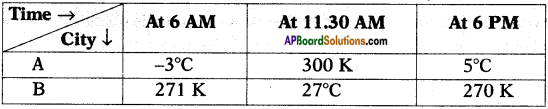On the basis of above table, answer the following questions.
1) In which city, the morning temperature at 6 O’clock is relatively high?
In ‘B’ city, the morning temperature at 6 O’clock is relatively high.

2) At what time, both cities are having the equal temperature?
At 11 : 30 AM, both cities are having the equal temperature.

Question 4.
A student took the same quantity of water and petrol in two different tumblers. He kept them on a table. When observed after a day there was water in a glass but petrol was completely evaporated. Give reasons why water was not evaporated completely but petrol was completely evaporated.
Petrol has a lower vapour point than water. And evaporation depends on the nature of the liquid so petrol evaporates quickly than water at room temperature.Question 5.
What are the differences between dew and fog?

 Dew Fog 1) Dew is the droplets that appear on the exposed objects in the morning or evening. 1) Fog is nothing but cloud on ground. 2) Dew does not effect visibility. 2) Due to fog visibility is greatly effected. 3) Dew is formed when relative humidity higher than temperature. 3) Fog is formed when island area is warmer than the ocean or large body of water.

Question 6.
Why is spirit evaporated in petri dish quickly under a fan when compared to that kept in closed room?

• The blowing air increases the rate of evaporation.
• This is because any molecule escaping from the surface is blown away from the vicinity of the liquid.
• This increases the rate of evaporation.
• This is the reason why the spirit in petri dish evaporates quickly when compared to that kept in closed room.

Question 7.
Does the temperature of water rise continuously if heat is supplied continuously?
Yes. If heat is supplied to water its temperature rises continuously till it reaches 100°C. At 100°C there would be no further rise of temperature, because the heat is sterilized to convert water to water vapour. So if heat is supplied beyond 100°C, all the water is converted into vapour.

Question 8.
Why does the mercury level of thermometer rise up when it is placed in hot water and fall down when it is placed in cold water?

• We know that bodies which are in contact achieve thermal equilibrium due to transfer of heat energy.
• If we keep thermometer in hot water, its mercury level rises because heat is transformed from hot body to cold body.
• Similarly, we observe that mercury level comes down when it is placed in cold water.

Question 9.
When we place thermometer in hot water, there is a rise in mercury level, thereafter it stops. What is the reason for steadiness of mercury level? What does reading of thermometer give at that time?

• The steadiness of the mercury column of the thermometer indicates that, flow of heat between the thermometer liquid (mercury) and water has stopped and thermal equilibrium has been attained between the water and thermometer liquid.
• The reading of thermometer gives thermal equilibrium state that is temperature.Question 10.
What is the relationship between temperature and kinetic energy?

• The average kinetic energy of molecules/particles of the hotter body is more than the colder body.
• So we can say that the temperature of a body is an indicator of the average kinetic energy of molecule of that body.
• So the average kinetic energy of molecules is directly proportional to the absolute temperature. [KE<sub>avg</sub> ∝ T]

Question 11.
What is the relationship between rise in temperature and specific heat of material?

• Temperature depends on nature of the material, hence the specific heat depends on its nature.
• If the specific heat, is high, the rise in temperature is low. [Q = mSΔt]
• It gives us an idea of degree of reluctance of a material to rise in temperature.

Question 12.
What is the principle of method of mixtures?
When two or more bodies at different temperatures are mixed with each other, then net heat lost by the hot bodies, is equal to net heat gained by the cold bodies until they attain thermal equilibrium or equal temperature.

Net heat lost = Net heat gained

This is known as principle of method of mixtures.

Question 13.
Why is evaporation of a liquid faster under a fan?

• If air is blown over the liquid surface in an open petri dish, a number of molecules evaporate from the surface of liquid.
• Because any molecule escaping from the surface is blown away from the vicinity of liquid.
• This increases the rate of evaporation.
• So evaporation of a liquid is faster under a fan.

Question 14.
Why do we get dew on the surface of a cold soft drink bottle kept in open air?

• The temperature of surrounding air is higher than the temperature of cold soft drink.
• Air contains water molecules in the form of water vapour which tend to condense by losing their kinetic energy to form water droplets.

Question 15.
Why does water take more time to become hot and take more time to become cool?
Water has the highest specific heat among all liquids. So it takes more time to become hot and takes more time to become cool.Question 16.
What is calorimeter? Name the material of which it is made of. Give two reasons for using the material stated by you.

• The vessel used for measurement of heat is calorimeter.
• It is made of thin sheet of copper.
• The reason is that the specific heat of copper is low and by making the vessel thin, its thermal capacity becomes low so that it takes a negligible amount of heat from its contents to attain the temperature of contents.

Question 17.
Why are burns caused by steam at 100°C more painful than that of water at 100°C?

• Water at 100°C takes additional heat energy to convert from liquid state to vapour (steam) state. This energy is called latent heat of vapourisation.
• Hence, steam at 100°C contains more heat energy than that of water at 100°C.
• So, burns caused by steam at 100°C are more painful than that of water at 100°C.

Question 18.
Why is cooking fast in a pressure cooker compared to open vessel?

• Boiling point of liquid increases with external pressure.
• Pressure cooker gives external pressure to the water in it.
• So, while cooking in pressure cooker it increases boiling point of water more than 100°C.
• So that, cooking is fast in pressure cooker compared to open vessel.

Question 19.
What happens to the water when wet clothes dry?
When wet clothes dry, the water molecules from wet clothes, after evaporation, change into water vapour and mix with water molecules present in surrounding air, in the form of water vapour.

Question 20.
Why do we use hot water bottles for fomentation?
The reason is that water does not cool quickly due to its large specific heat, so hot water bottle provides heat energy for fomentation for a long time.

Question 21.
Why do all plants and animals have a high content of water in their bodies?
All plants and animals have nearly 80% to 90% of water in their bodies. So it helps in maintaining the body temperature in all seasons due to high specific heat.

Question 22.
Water is used as an effective coolant. Give reason.
1) Water is used as an effective coolant because by allowing water to flow in pipes around the heated parts of machine, heat energy from such parts is removed (e.g. radiators in car and generator are filled with water).
2) Water in pipes extracts more heat from surroundings without much rise in its temperature because of its large specific heat.

Question 23.
Why is the base of cooking pan made thick?

• By making the base of cooking pan thick, its thermal capacity becomes large and it imparts sufficient heat energy at a lower temperature to the food for its proper cooking.
• Further, it keeps the food warm for a long time, after cooking.

Question 24.
Water in lakes and ponds in cold countries does not freeze all at once. Give reason.

• The latent heat of fusion of ice is sufficiently high.
• So to freeze water, a large quantity of heat has to be withdrawn, hence it freezes slowly and thus keeps the surroundings moderate.Question 25.
Why do drinks get more quickly cooled by adding pieces of ice at 0°C than ice-cold water at 0°C?

• This is because 1 g of ice at 0°C takes 336 J of heat energy from the drink to melt into water at 0°C.
• Thus drink loses an additional 336 J of heat energy for 1 g of ice at 0°C than for 1 g ice cold water at 0°C. Therefore cooling produced by 1 g of ice at 0°C is more than that by 1 g of water at 0°C.

Question 26.
When ice in a frozen lake starts melting, its surroundings become very cold? Why?
The reason is that the heat energy required for melting the frozen lake is absorbed from the surrounding atmosphere. As a result, the temperature of surroundings falls and it becomes very cold.

Question 27.
Why is it more cold after the hail-storm than during or before the hail-storm?
The reason is that after the hail-storm, the ice absorbs the heat energy required for melting from the surroundings, so the temperature of the surroundings falls further down and we feel more cold.

Question 28.
Which of the substances A, B, and C has the least specific heat? The temperature versus time graph as shown below.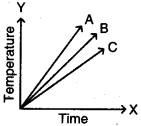The substance ‘A’ has least specific heat because the rise in temperature is more for substance. We know that specific heat and rise in temperature are inversely proportional. So A has the least specific heat.

Question 29.
Why do we need pressure cooker to cook food at higher altitudes?

• At higher altitudes, such as hills and mountains, atmospheric pressure is low, therefore water boils at a temperature lower than 100°C and so it does not provide the required heat energy for cooking.
• Thus cooking there becomes very difficult and it takes a much longer time.
• So we require a pressure cooker to cook the food at a faster rate.

Question 30.
Lalitha wants to determine the specific heat of Aluminium shots. What apparatus of material is required to do this experiment?
The apparatus required is calorie meter, thermometer, stirrer, water, steam heater, wooden box, and aluminium shots.Question 31.
What are the material required in order to find specific heat of soild?
Calorimeter, thermometer, stirrer, water, steam, heater, wooden box, and lead shots.

Question 32.
Your teacher made an experiment to show the formation of dew and frost. Explain how you show the formation of dew and frost.
Place a water bottle in a deep fridge of refrigerator. After some time remove bottle from the refrigerator. We can observe ice in the bottle and water droplets are formed outside the bottle. This experiment is useful in formation of dew and frost.

Question 33.
Why a bottle completely filled with water and closed with a tight cap break after freezing?

• Density of ice is less than that of water.
• This means water expands on freezing and converts into ice.
• So, excess space is required to expand the water.
• The bottle completely filled with water and closed with a tight cap has no excess space to expand water.
• So, bottle breaks while freezing water in it.

Question 34.
Which of the following substances take more time to raise its temperature for a certain degree Celsius? Give reason.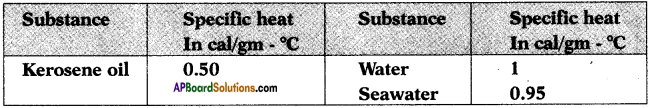Water takes more time to raise temperature because it has greater specific heat is, i. e., 1 cal/g-°C. As the specific heat of substance increases, it takes more time to raise its temperature.

Question 35.
The graph shows variations of temperature (T) of one kilogram of material with the heat (H) supplied to it.
At ‘O’ the substance is in the solid state. From the graph can conclude that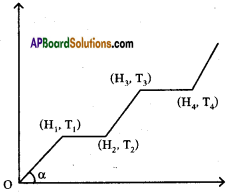i) The melting point of the solid is …………….
The melting point of the solid is T1

ii) The latent heat of fusion is …………….
The latent heat of fusion is (H2 – H1)

iii) The latent heat of vaporisation is …………….
The latent heat of vaporisation is (H4 – H3)

iv) The boiling point of the substance is ……………
The boiling point of the substance is T3

Question 36.
Which of the substances A, B, and C has the highest specific heat? The temperature versus time graph as shown given below.• The substance ‘C’ has the highest specific heat.
• Because ‘C’ does not rise its temperature quickly. In other words ‘C’ takes more time to rise its temperature.

Question 37.
Can the average kinetic energy of a body be even zero?
The average kinetic energy of a body can be zero.
Reason :
K.E = $$\frac{1}{2}$$ mv2
m is never zero
if v = 0; then body is not in the motion.

Question 38.
A slab of ice at -50°C is constantly heated till the steam attains a temperature of 150°C. Draw a graph showing the change in temperature with time. Label the various parts of the graph properly.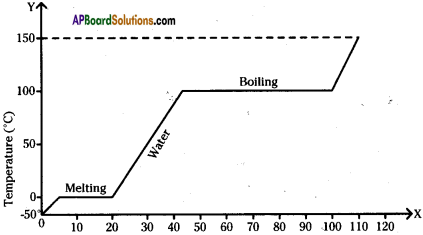Question 39.
Iron of weight 2 kg was supplied with 12000 calories of heat. Initial temperature of iron was 20°C. Its specific heat is 0.1 cal/g-°C. What is the final temperature of iron?
Mass of iron (m) = 2 kg = 2 × 1000 g. = 2000 g.
Quantity of heat supplied (Q) = 12,000 cal.
Initial temperature = θ(i) = 20°C
Final temperature = θf = ?
Specific heat of iron (s) = 0.1 cal / g / °C.
Heat = Q = ms∆θ
Q = ms (θf – θi)
θf – θi = Q/ms
θf – 20 = $$\frac{12000}{2000 \times 0.1}=\frac{12}{2 \times 0.1}$$ = 60
θf = 60 + 20 = 80°C
∴ The final temperature of iron = θf = 80°CQuestion 40.
What is the heat energy required to rise 20 kg of water from 25° C to 75° C?
Given m = 20 kg = 20,000 gm
t1 = 25° C ; t2 = 75° C ;
S = 1 cal/gm °C.
Q = mS∆T = 20000 × 1 × (75 – 25) = 20000 × 50
Q = 1000000 calories

Question 41.
If you drink 200 ml of water at 20° C, what is the heat gained by water from your body? (Body temperature is 37° C)
m = 200 g (1 ml of water = 1 gm of water)
S = 1 cal/gm °C ;
t1 = 20° C; t2 = 37° C
Q = mS∆T (∆T = t2 – t1)
= 200 × 1 × (37 – 20)
= 200 × 17
Q = 3400 calories

Question 42.
What would be the final temperature of a mixture of 60 gm of water at 30°C temperature and 60 gm of water at 60°C temperature?
m1 = 60 g ;
T1 = 30°C ;
m2 = 60 g ;
T2 = 60°C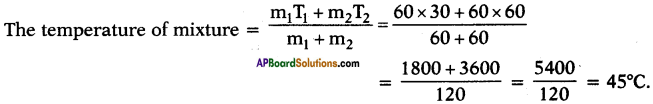Question 43.
The quantity of heat which can rise the temperature of ‘x’ gram of a substance through t1°C and the quantity of heat which can rise the temperature of ‘y’ grams of water through t2°C is same. What is ratio of specific heats? What is ratio of specific heats if rise in temperatures are same and if amount of substances are same?
Suppose specific heats of substance and water are s1 and s2 respectively.
Heat absorbed by x gram of substance to rise its temperature to t1°C.
Q1 = ms∆T = x × s1 × t1
Heat absorbed by y gram of water to rise its temperature to t2°C.
Q2 = ms∆T2 = y × s2 × t2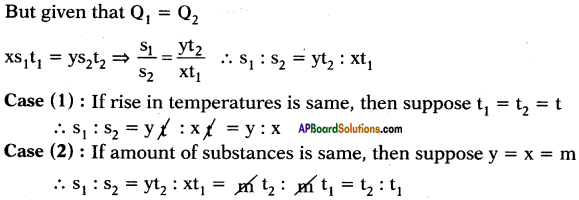### 10th Class Physics 1st Lesson Heat 4 Marks Important Questions and Answers

Question 1.
Write the factors that effect the process of evaporation. Explain with suitable examples. (AP March 2017)
Process of evaporation is effected by surface area, wind speed, humidity, and temperature.
Ex:

• The water kept in a china dish evaporates faster than in a cup because of more surface area.
• Water in wet the clothes are kept under fan evaporates faster than in normal conditions.
• Water in wet clothes evaporates faster on a less humid day than on a more humid day.

Question 2.
A) Write the principle of method of Mixtures.
B) What would be the final temperature of a mixture of 60 gms of water at 50°C and 50 gms of water at 70°C? (AP March 2018)
A) Principle of method of mixtures :
Net heat lost by the hot body = Net heat gain by the cold body.

B) m1 = 60 gms.,
T1 = 50°C ;
m2 = 50 gms.,
T2 = 70°C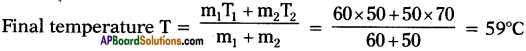Question 3.
Answer the following questions by using the data given in the table. (AP March 2018)

 Substance Specific heat (cal / g°C) Lead 0.031 Aluminium 0.21 Copper 0.095 Water 1.00 Iron 0.115

a) Write SI units for specific heat.
Joule / Kilogram-Kelvin

b) Based on specific heat values, arrange the substances given in the table in ascending order.

c) If we supply same quantity of heat, which substance will heat up faster?

d) Calculate the amount of heat required to raise the temperature of 1 kg of Iron through 10°C.
Q = mS∆T = 1000 × 0.115 × 10 = 1150 cal.Question 4.
Suggest an experiment to show that when ice is converted into water, its temperature does not change. How much heat is required to convert 5 grams of ice at 0°C to water, at the same temperature? (Latent heat of fusion of ice is 80 cal/gram). (TS June 2015)
Procedure :

1. Take small ice cube in a beaker. Insert the thermometer in the beaker.
2. Now start heating the beaker and note down readings of thermometer every one minute till the ice completely melts and gets converted into water.
3. Before heating the temperature of ice is 0° C or less than 0° C.

Observation :

1. We will observe that the temperature of ice at the beginning is equal to or below 0°C.
2. If the temperature of ice is below 0°C, it goes on changing till it reaches 0° C.
3. When ice starts melting, we will observe no change in temperature though you are supplying heat continuously.

Explanation :

1. Given heat energy is used to break the bonds (H2O) in ice and melts.
2. So, temperature is constant while melting.

Conclusion :

1. This process is called melting. In this process heat converts solid phase to liquid phase.
2. The temperature of the substance does not change until all the ice melts and converts into water.
3. The heat given to melting is called latent heat of fusion.
4. The heat required to convert 1 gm of solid completely to liquid at constant temperature is called “latent heat of fusion”.
m = 5 gm; Lf = 80 cal/g

The amount of heat absorbed Q = MLf = 5 × 80 = 400 cal /g.

Question 5.
The graph shows the values of temperature, when ice is heated till it becomes water vapour. Observe the graph and answer the following questions. (TS March 2016)
(Note that the figure is not completely quantitative and also not to the scale. It is purely qualitative)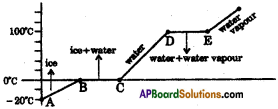a) At what temperature, ice converts into water?
Ice converts into water at 0°C and above.

b) What does $$\overline{\mathrm{DE}}$$ represent?
$$\overline{\mathrm{DE}}$$ represents the latent heat of vapourisation.

c) What is the range of temperature of liquid water?
The range of temperature of liquid water is 0°C to 100°C

d) Which part of the graph represents change of state from ice to water?
$$\overline{\mathrm{BC}}$$ represents the change of state of ice to water.

Question 6.
Write the differences between heat and temperature.

 Heat Temperature 1) It is a thermal energy. 1) It is the measurement of hotness or coldness. 2) Heat is an extensive property, means it does not depend on amount of the substance that is present. 2) Temperature is an intensive property means that the substance present will not change the specific characteristic. 3) Heat is the amount of energy of the system. 3) Temperature is the measure of the average molecular motions in a system. 4) Its S.I unit is Joules. 4) Its S.I. unit is degrees C or K. 5) Heat is energy itself that flows. 5) It decides the direction of heat flow.

Question 7.
Why is the specific heat different for different substances?

• We know that the temperature of a body is directly proportional to the average kinetic energy of particles of the body.
• The molecules of the system have different forms of energies. The total energy of the system is called internal energy of the system.
• When we supply heat energy to the system, the heat energy given to it will be shared by the molecules among the various forms of energy.
• This sharing will vary from substance to substance.
• The rise in temperature is high for a substance, if the maximum share of heat energy is utilised for its linear K.E.
• This sharing of heat energy also varies with temperature. That is why the specific heat is different for different substances.Question 8.
Explain the process of evaporation.

• The molecules of a liquid that kept in a dish, continuously move with random speeds in various directions. As a result, these molecules collide with other molecules.
• During this collision they transfer energy to other molecules. Hence, the molecules at the surface acquire energy and may fly off from the surface.
• Some of these escaping molecules may be directed back into liquid when they collide with the particles of air.
• If the number of escaping molecules is greater than the number returned, then the number of molecules in the liquid decreases.
• Thus when a liquid is exposed to air, the molecules at the surface keep on escaping from the surface till the entire liquid disappears into air. This process is called evaporation.

Question 9.
Define evaporation. Explain what are the affecting factors of evaporation and how they effect the rate of evaporation.
Evaporation :
The process of escaping of molecules from the surface of liquid at any temperature is called evaporation.

The affecting factors of evaporation :

1. Temperature,
2. Surface area,
3. Wind speed,
4. Humidity.

The affection on the rate of evaporation :
1) Temperature :
As the temperature increases evaporation increases.

2) Surface area :
As the surface area of liquid increases, more molecules tend to leave the surface. So rate of evaporation increases.

3) Wind speed :
As the wind speed increases rate of evaporation increases.

4) Humidity :
As the humidity increases rate of evaporation decreases.

Question 10.
Why is climate near the seashore moderate?

1. The specific heat of water is very high. It is about five times as that of sand.
2. Hence the heat energy required for the same rise in temperature by certain mass pf water will be nearly five times than that required by same mass of sand.
3. Similarly, a certain mass of water will give out nearly five times more heat energy than that given by sand of the same mass for the same fall in temperature.
4. As such, sand (or earth) gets heated or cooled more rapidly as compared to water under similar conditions.
5. Thus, a large difference in temperature is developed between the land and sea due to which land and sea breezes are formed.
6. These breezes make the climate near seashore moderate.

Question 10.
Why do farmers fill their fields with water on a cold winter night?

• In the absence of water, if on a cold winter night, the atmospheric temperature falls below 0°C, the water in the fine capillaries of plants will freeze, so the veins will burst due to the increase in volume of water on freezing.
• As a result, plants will die and the crop will be destroyed.
• In order to save crop on such cold nights, farmers fill their field with water because water has high specific heat, so it does not allow the temperature in the plants of surrounding area to fall up to 0°C.Question 11.
Explain the factors effecting boiling.
The factors effecting boiling are
I) Pressure :

1. The boiling point of pure water at one atmospheric pressure is 100°C.
2. Water boils at a temperature higher than 100°C, if the atmospheric pressure is higher than one atmosphere pressure, and boils at a temperature lower than 100°C, if the atmospheric pressure is less than 1 atmosphere.

II) Impurities :
The boiling point of liquid increases by the addition of impurities to it. If a little common salt is added to water, the water boils at a temperature higher than 100°C.

Question 12.
A, B and C are the three liquids at 20°C, 30°C and 40°C respectively. If equal masses of A and B are mixed, the resultant temperature is 26°C. If equal masses of A and C are mixed, the resultant temperature is 33°C. Find the ratio of specific heats of A, B and C.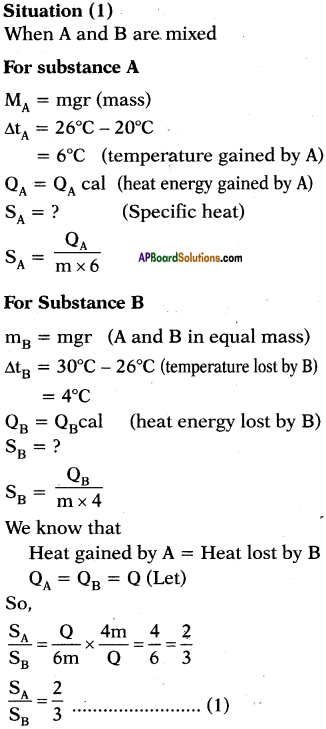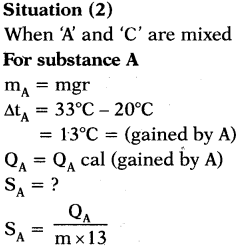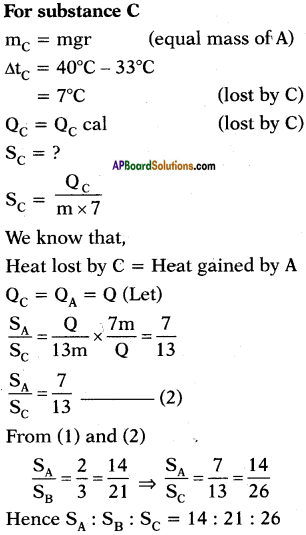Question 13.
A refrigerator converts 5kg of water at 40°C into ice at 0°C in 20 minutes. Find the power of refrigerator.
m = 5kg = 5000gr
∆t = 40°C – 0°C = 40°C
S = 1 (water) .
Q = m.s.∆t = 5000.1.40 = 200000 cal.
We know the relation between heat and work done as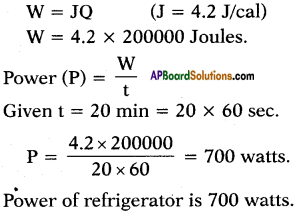Question 14.
Snow on mountains does not melt all at once. Why?

• Snow on mountains does not melt all at once because the ice has a high specific latent heat of fusion.
• It is due to this fact that it changes into water slowly as it gets heat energy from the sun.
• If latent heat would not have been so high, the snow would have melted quickly even with a small amount of heat energy and there would have been floods in rivers.

Question 15.
Collect specific heats of various substances.

 Substance Specific heat In cal/g – °C In J/kg – K Lead 0.031 130 Mercury 0.033 139 Brass 0.092 380 Zinc 0.093 391 Copper 0.095 399 Iron 0.115 483 Glass(flint) 0.12 504 Aluminium 0.21 882 Kerosene oil 0.50 2100 Ice 0.50 2100 Water 1 4180 Sea water 0.95 3900

Question 16.
The graph given below represents a cooling curve for a substance being cooled from a higher temperature to a lower temperature.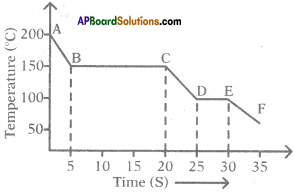a) What is the boiling point of the substance?
The boiling point of the substance is 150°C (because the part BC represents condensation where the vapour changes into the liquid without the change in temperature).

b) What happens in the region DE?
The region DE represents freezing of the substance where the liquid changes into solid at a constant temperature that is 100°C.

c) What is the melting point of the substance?
The melting point of substance is 100°C.

Question 17.
You’ve taken water in vessel at 0°C and closed it with a glass vessel as shown in the figure. You used and created a vacuum inside.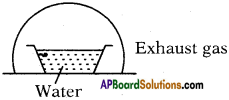a) Explain what happens.
b) A part of water condenses; what is the amount of water that gest condensed?
a) At 0°C also water is available in liquid state (generally at 0°C ice is also available) because the air in vacuum rise the temperature. Here evacuation is possible so it allows evaporation.

b) Let y ml of water is taken at 0°C.
’x’ ml of water is evaporated
Latent heat of vapourisation = Lsteam = 540 Cal/g.
Latent heat of ice = Lice = 80 Cal/g.
After sometime conversion process stops. So equilibrium is possible.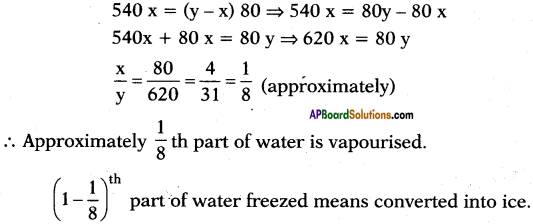Question 18.
What are the applications of specific heat capacity?
1. The oceans behave like heat store houses for the earth. They absorb large amounts of heat at the equator without rise in temperature due to high specific heat capacity of water. So, oceans moderate the surrounding temperature near the equator. Ocean water transports the heat away from the equator to areas closer to the north and south poles. This transported heat helps moderate the climates in parts of the Earth that are far from the equator.

2. Watermelon brought out from the refrigerator retains its coolness for a long time than any other fruit because it contains a large amount of water (water has greater specific heat).

3. The samosa seems to be cool outside but it is hot when we eat it because the curry inside the samosa contains ingredients with higher specific heats.Question 19.
Some hot water Is added to three times its mass of cold water at 10°C. The resulting temperature is found to be 20°C. Find the initial temperature of hot water.
Let the initial temperature of hot water be t°C.
Mass of hot water = mg
Mass of cold water = 3 mg
Initial temperature of cold water = 10°C
And resultant temperature = 20°C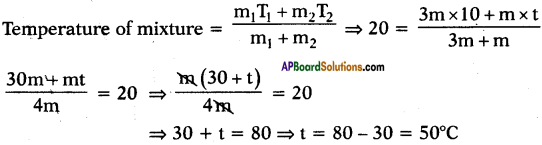∴ Initial temperature of hot water = 50°C.

Question 20.
40 g of water at 60°C is poured into vessel of 200 g mass containing 50 g of water at 20°C. The final temperature of mixture is 30°C. Calculate the specific heat of vessel.
Mass of hot water at 60°C = 40 g
Mass of cold water at 20°C = 50 g
Mass of vessel = 200 g
Suppose the specific heat of vessel is Sv
Heat energy given by hot water = mSw∆T
= 40 × 1 × (60 – 30) [∵ T1 = 60°C, T2 = 30°C]
= 40 × 30 = 1200 cal
Heat energy taken by cold water = mSw∆T
= 50 × 1 × (30 – 20) = 50 × 10 = 500 cal
Heat energy taken by vessel = mSv∆T = 200 × Sv × (30 – 20) = 2000 Sv
According to the principle of method of mixtures,
Heat lost by hot water = heat gained by cold water + heat gained by vessel 1200 = 500 + 2000 Sv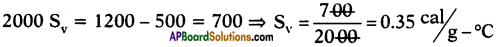Question 21.
A, B and C are three liquids at 20°C, 30°C and 40°C respectively. If equal masses of A and B are mixed, the resultant temperature is 29°C. The equal masses of A and C are mixed, the resultant temperature is 33°C. Find the specific heats of A, B and C.
Suppose specific heats of liquids A, B and C are s1, s2 and s3 respectively.
Given that the temperatures of liquids are 20°C, 30°C and 40°C.
Given that equal mass of A and B are mixed, the resultant temperature is 29°C.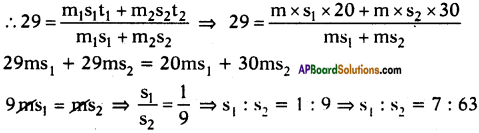Further given that equal masses of A and C are mixed, the resultant temperature is 33°C.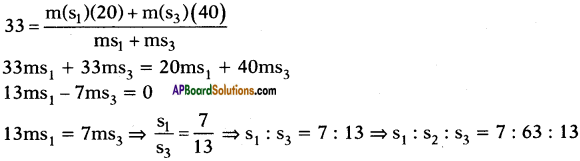Question 22.
A refrigerator converts 5 kg of water at 40°C into ice at 0°C. Find the total energy released in Joules.
m = 5 kg = 5000 g
The heat energy released to convert 5 kg of water at 40°C to 5 kg of water at 0°C.
Q1 = ms∆T
= 5000 × 1 × (40 – 0) = 5000 × 40 = 200000 cal [s = 1 cal/g-°C for water]
The heat energy released to convert 5 kg of water at 0°C to 5 kg of ice at 0°C.
Q2 = mLf
= 5000 × 80 = 400000 cal [∵ Lf = 80 cal/g]
Total energy released = 200000 + 400000
= 600000 cal
= 600 kcal
= 142.86 kJ.

Question 23.
The quantity of heat which can rise the temperature ‘x’ grams of a substance through t1°C can rise the temperature of ‘y’ grams of water through t2°C is same.
What is the ratio of specific heats?